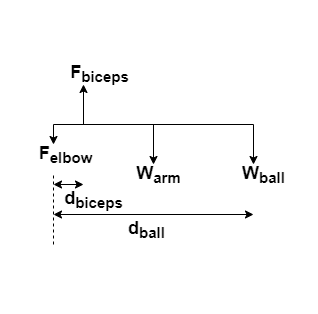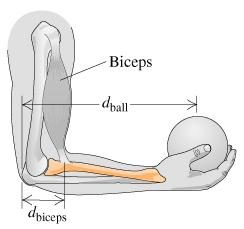# Problem: When you lift an object by moving only your forearm, the main lifting muscle in your arm is the biceps. Suppose the mass of a forearm is 1.00 kg. If the biceps is connected to the forearm a distance dbiceps = 3.50 cm from the elbow, how much force Fbiceps must the biceps exert to hold a 600 g ball at the end of the forearm at distance dball = 37.0 cm from the elbow, with the forearm parallel to the floor?PREPAREStart by modeling the forearm as a simple shape. Draw a visual overview showing all the forces and distances. List the known information and identify what must be found. Pick an axis about which to calculate the torques and determine the torque about this point due to each force acting on the forearm.

###### FREE Expert Solution

Torque:

$\overline{){\mathbf{\tau }}{\mathbf{=}}{\mathbf{r}}{\mathbf{·}}{\mathbf{F}}}$darm = dball/2

81% (286 ratings)###### Problem Details

When you lift an object by moving only your forearm, the main lifting muscle in your arm is the biceps. Suppose the mass of a forearm is 1.00 kg. If the biceps is connected to the forearm a distance dbiceps = 3.50 cm from the elbow, how much force Fbiceps must the biceps exert to hold a 600 g ball at the end of the forearm at distance dball = 37.0 cm from the elbow, with the forearm parallel to the floor?PREPARE

Start by modeling the forearm as a simple shape. Draw a visual overview showing all the forces and distances. List the known information and identify what must be found. Pick an axis about which to calculate the torques and determine the torque about this point due to each force acting on the forearm.